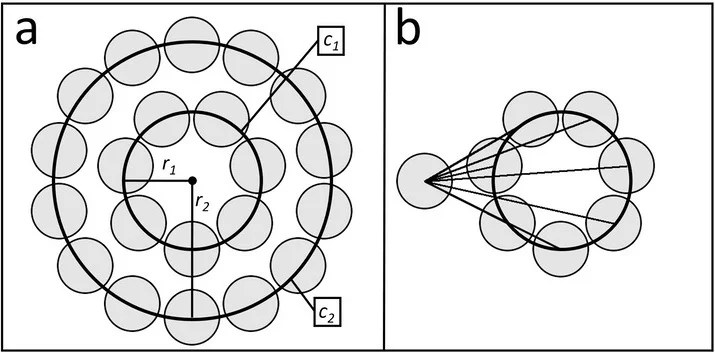# Mercury (Not Venus) Is The Closest Planet To Earth | New Study

• The new point-circle method accurately calculates the distance (averaged over time) between two planets.
• It shows that Mercury is the nearest neighbor to all 7 other planets in the solar system, including Earth.

In school, you have been taught that the closest planet to Earth is Venus. In fact, if you Google right now, you will probably find the same answer. However, this is not entirely correct: Venus isn’t the nearest when averaged over time.

According to the NASA literature, Venus is our closest planetary neighbor, which is true in a sense that Venus has the closest approach to Earth. However, the story becomes a lot more interesting if you consider the average distance between any two planets.

Recently, researchers at the University of Alabama and Los Alamos National Laboratory devised a mathematical model to accurately determine the distance (averaged over time) between two planets. The model shows that Mercury is the closest planet to Earth, as well as to six other planets in the solar system.

### The Mathematical Method

The traditional method assumes that all planets are in coplanar orbits and calculates the distance between two planets by subtracting the average radius of the inner orbit from the average radius of the outer orbit. This yields only the average gap of the nearest points.

The new point-circle method, on the other hand, considers the orbits of two astronomical bodies as coplanar, circular and concentric. The position of a planet at any specific time can be considered a probabilistic distribution of its orbit (as shown in figure 1a).Figure 1a and 1b

Thus, an average distance between two bodies can be determined by calculating the average distance of each point on the outer circle (c2) to each point on the inner circle (c1). But since the planet’s structure is rotationally symmetric, the average distance from any given point on the outer circle to every point on the inner circle is the same (figure 1b).

The method shows that the distance (averaged over time) between any two orbiting objects is proportional to the inner orbits’ relative radius. Since Venus orbits the Sun more closely (on average), it’s more distant to Earth than Mercury.

The team applied this method on all planets in our solar system and found that Mercury is the closest planet to all 7 other planets. The average distance between Mercury and Earth is 1.038 AU, whereas the average distance between Venus and Earth is 1.136 AU.

### Validating ResultsComparison of average distances (in AU) between planets, obtained via different methods | Courtesy of researchers

To confirm the results, researchers ran a computer simulation that mapped locations of all 8 planets for 10,000 years. After one day, the software recorded the distances between every single pair of planets in our solar system.

This model can be applied to any two bodies that have concentric, circular and coplanar orbits. It can be used to rapidly calculate the satellite communication relays, in which signal strength decreases significantly with the square of distance.Written by
###### Varun Kumar

Varun Kumar is a professional science and technology journalist and a big fan of AI, machines, and space exploration. He received a Master's degree in computer science from GGSIPU University. To find out about his latest projects, feel free to directly email him at [email protected]

•Cristobaldelicia says:
•Varun Kumar says: